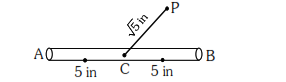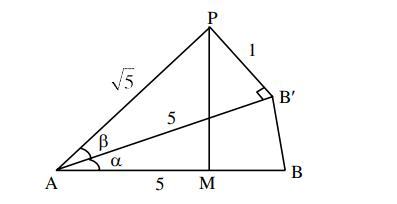# A 10 inches long pencil AB with mid point C and a small eraser P are placed on the horizontal top`
Question:

A 10 inches long pencil $\mathrm{AB}$ with mid point $\mathrm{C}$ and a small eraser P are placed on the horizontal top of a table such that $\quad \mathrm{PC}=\sqrt{5} \quad$ inches and $\angle \mathrm{PCB}=\tan ^{-1}(2)$. The acute angle through which the pencil must be rotated about $\mathrm{C}$ so that the perpendicular distance between eraser and pencil becomes exactly 1 inch is:1. $\tan ^{-1}\left(\frac{3}{4}\right)$

2. $\tan ^{-1}(1)$

3. $\tan ^{-1}\left(\frac{4}{3}\right)$

4. $\tan ^{-1}\left(\frac{1}{2}\right)$

Correct Option: 1

Solution:From figure.

$\sin \beta=\frac{1}{\sqrt{5}}$

$\therefore \tan \beta=\frac{1}{2}$

$\tan (\alpha+\beta)=2$

$\frac{\tan \alpha+\frac{1}{2}}{1-\tan \alpha\left(\frac{1}{2}\right)}=2$

$\tan \alpha=\frac{3}{4}$

$\alpha=\tan ^{1}\left(\frac{3}{4}\right)$